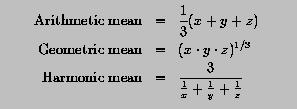# Programming Example: Computing Means### Problem Statement

Given three real numbers, its arithmetic mean (average), geometric mean and harmonic mean are defined as follows:Write a program to compute and display the means of three REAL variables initialized with positive real values.### Solution

```! -------------------------------------------------------
!   Computes arithmetic, geometric and harmonic means
! -------------------------------------------------------

PROGRAM  ComputeMeans
IMPLICIT  NONE

REAL  :: X = 1.0, Y = 2.0, Z = 3.0
REAL  :: ArithMean, GeoMean, HarmMean

WRITE(*,*)  'Data items: ', X, Y, Z
WRITE(*,*)

ArithMean = (X + Y + Z)/3.0
GeoMean   = (X * Y * Z)**(1.0/3.0)
HarmMean  = 3.0/(1.0/X + 1.0/Y + 1.0/Z)

WRITE(*,*)  'Arithmetic mean = ', ArithMean
WRITE(*,*)  'Geometric mean  = ', GeoMean
WRITE(*,*)  'Harmonic Mean   = ', HarmMean

END PROGRAM ComputeMeans
```
Click here to download this program.### Program Output

``` Data items: 1.,  2.,  3.

Arithmetic mean = 2.
Geometric mean  = 1.81712067
Harmonic Mean   = 1.63636363
```### Discussion

• Variables X, Y and Z are initialized in the first REAL statement, while the second declares three variables, ArithMean, GeoMean and HarmMean, for holding the result.
• The first WRITE statement displays the values of X, Y and Z. The second WRITE generates a blank line.
• In the second assignment statement that computes the geometric mean, the exponent part is 1.0/3.0 instead of 1/3, since the latter is zero. 1.0/3 and 1.0/3 also work fine. But, you should not use 0.3, since it is not equal to 1/3.

The parenthesis surrounding X * Y * Z cannot be removed; otherwise, the expression X * Y * Z **(1.0/3.0) means X * Y * (Z **(1.0/3.0)) since ** has a priority higher than that of *.

• The parenthesis in the third assignment cannot be removed either. Why?